## A carousel has a diameter of 6.0-m and completes one rotation every 1.7s. Find the centripetal acceleration of the traveler in m / s2.

Question

A carousel has a diameter of 6.0-m and completes one rotation every 1.7s. Find the centripetal acceleration of the traveler in m / s2.

in progress 0
6 months 2021-08-31T10:39:03+00:00 1 Answers 0 views 0

The centripetal acceleration of the traveler is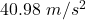Explanation:

It is given that, A carousel has a diameter of 6.0-m and completes one rotation every 1.7 s.

We need to find the centripetal acceleration of the traveler. It is given by the formula as follows :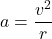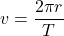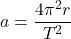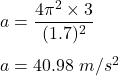So, the centripetal acceleration of the traveler is.